Anzeige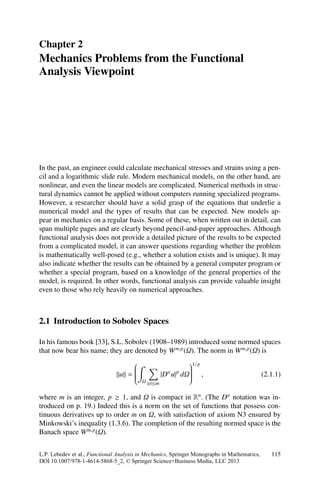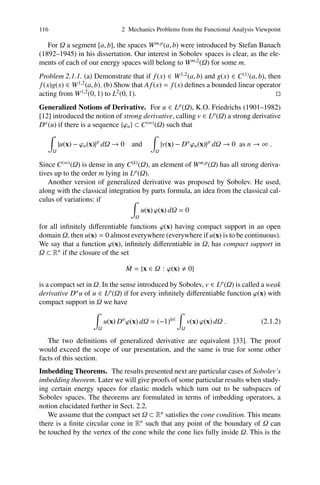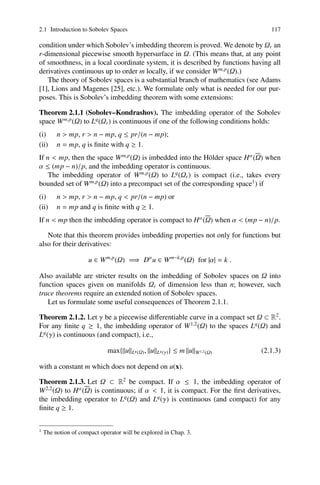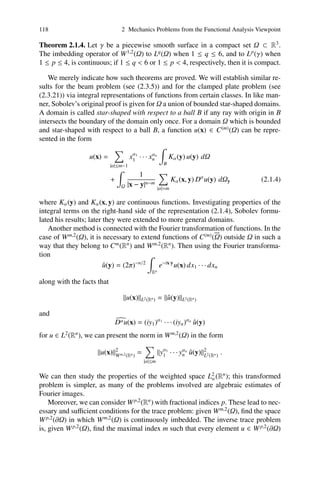Anzeige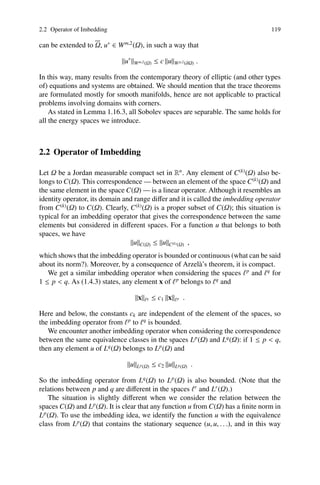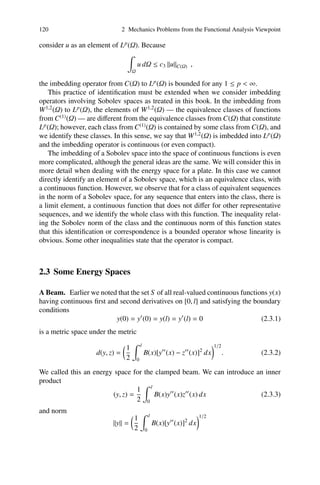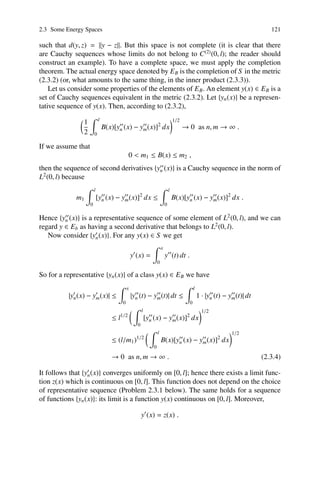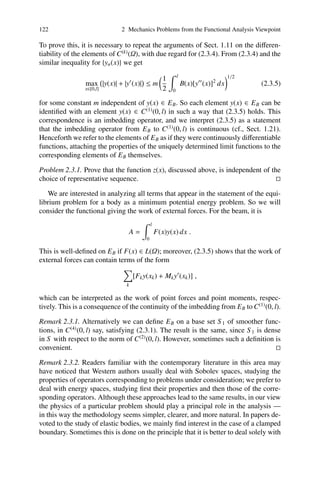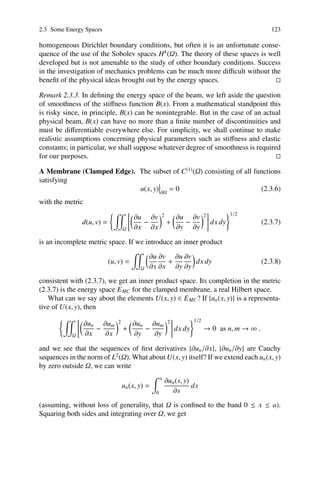Anzeige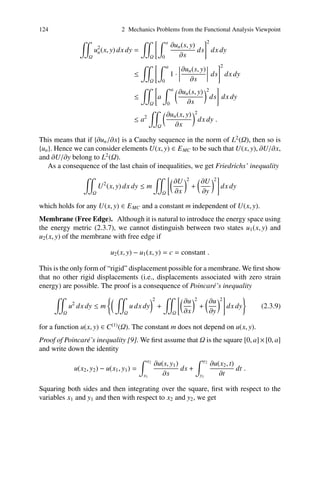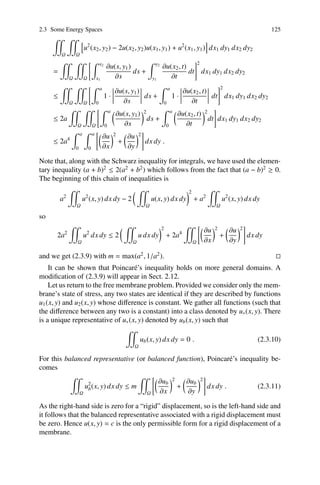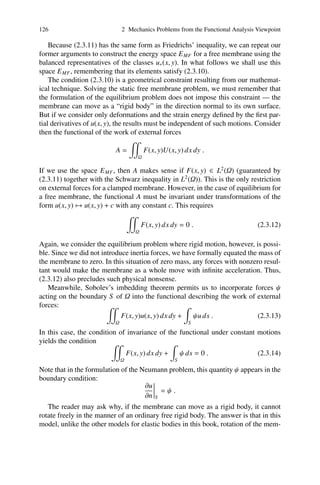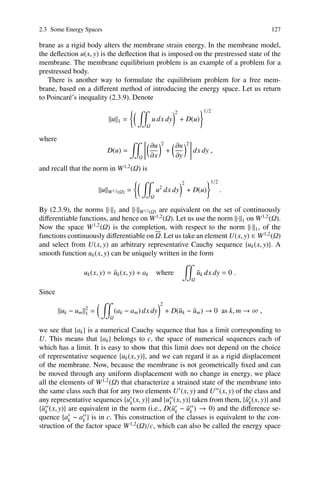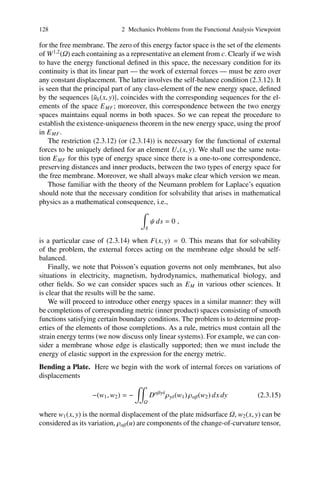Anzeige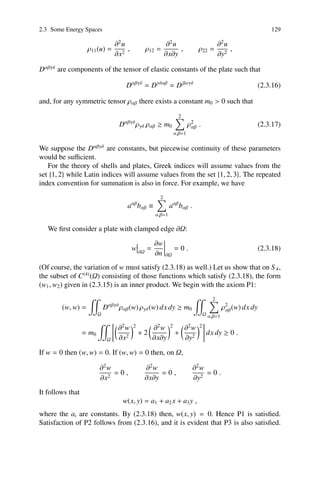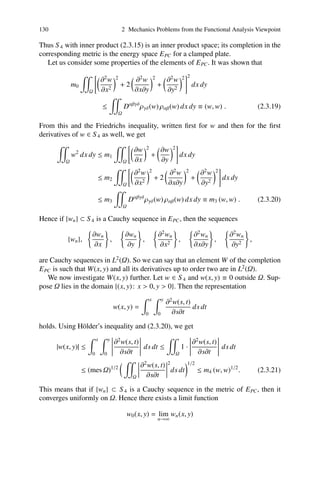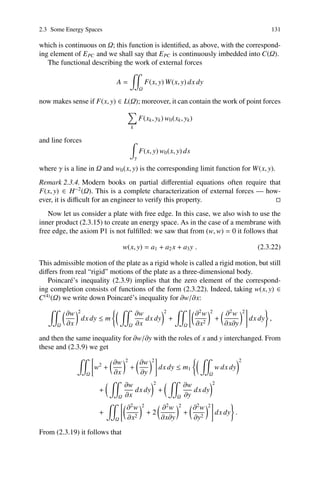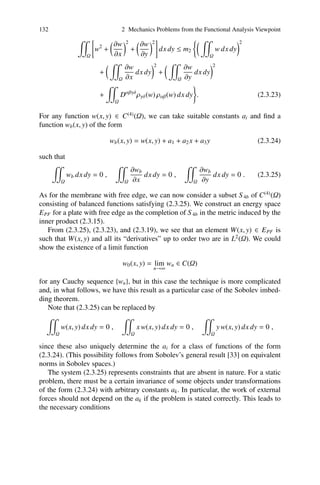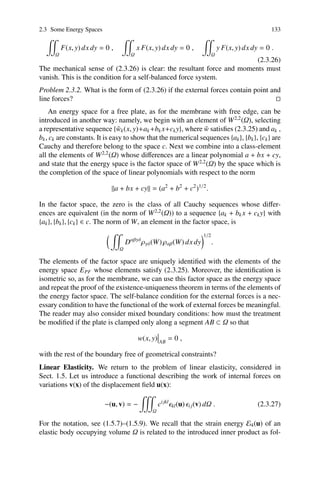Anzeige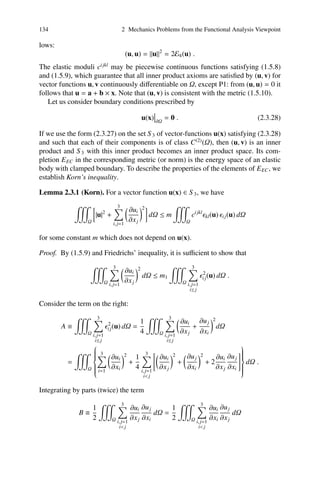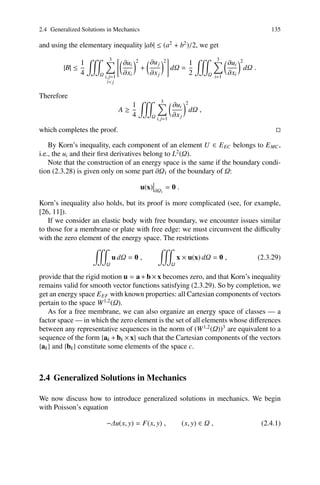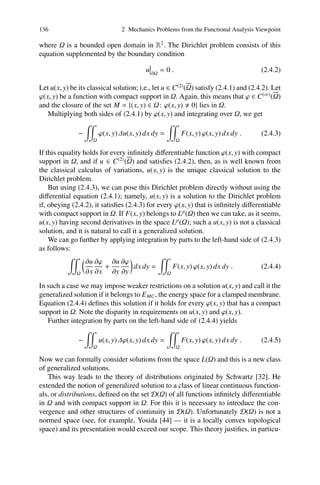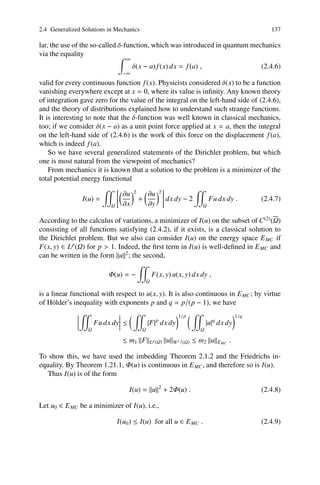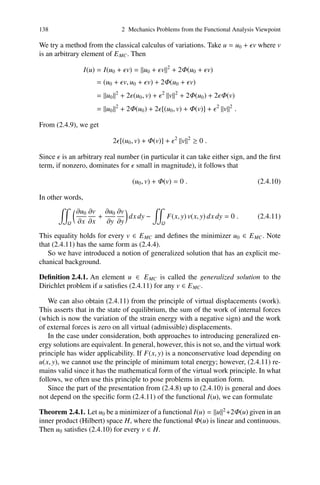Anzeige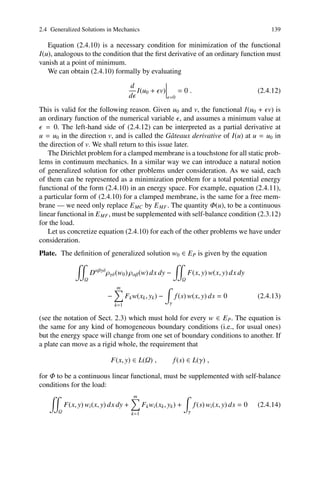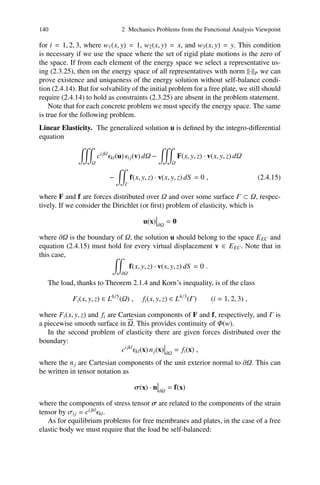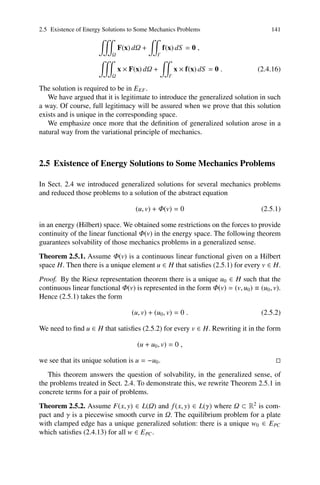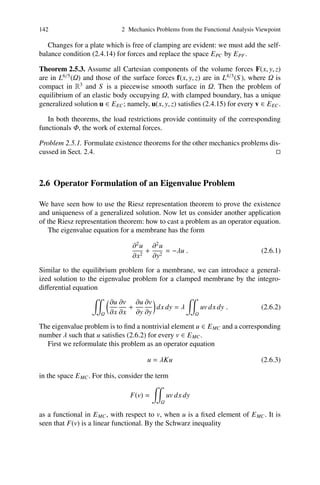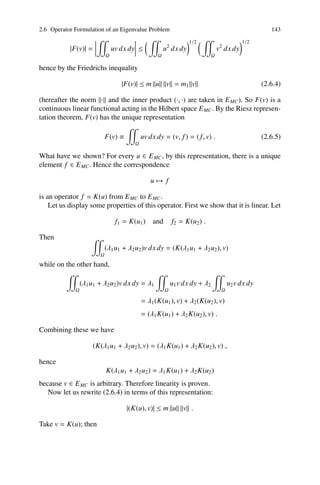Anzeige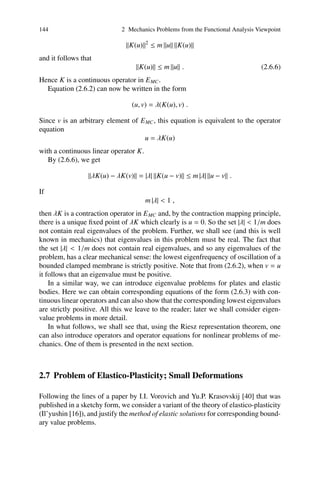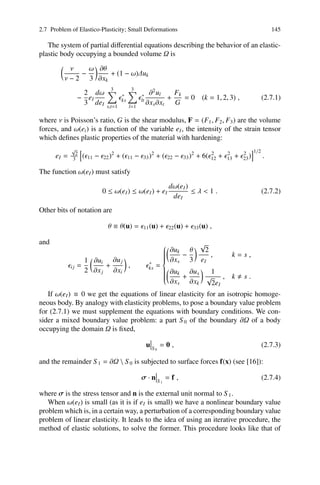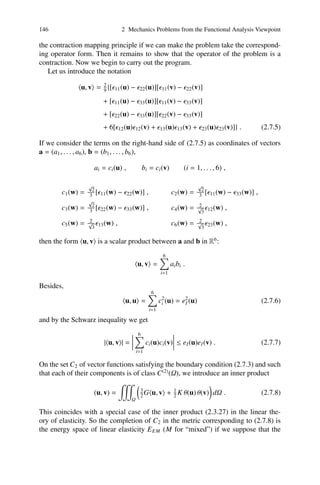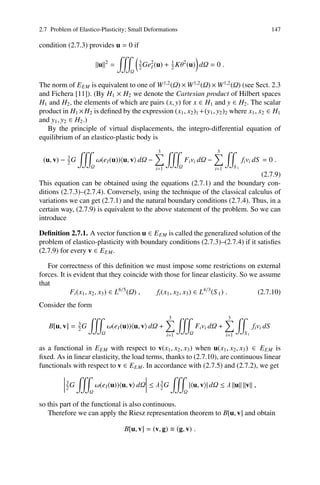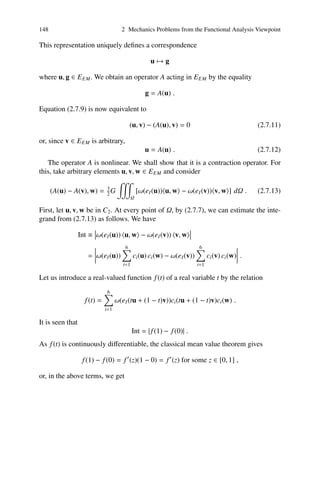Anzeige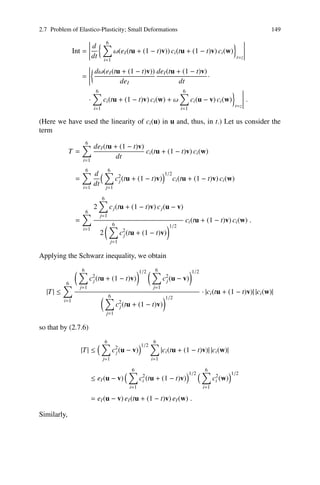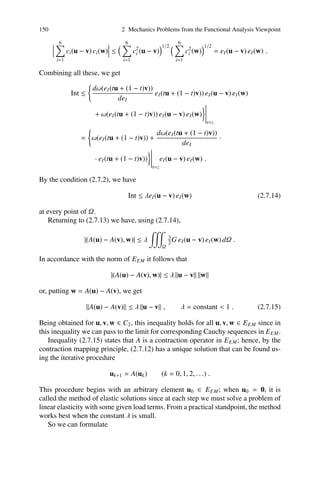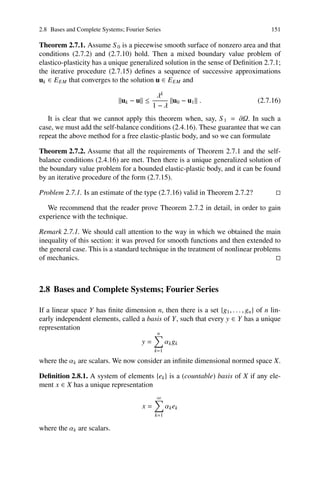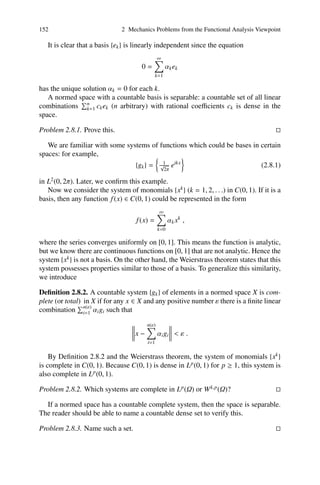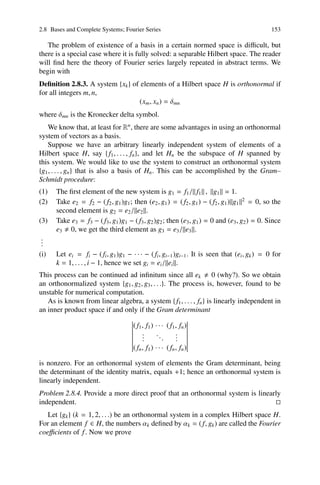Anzeige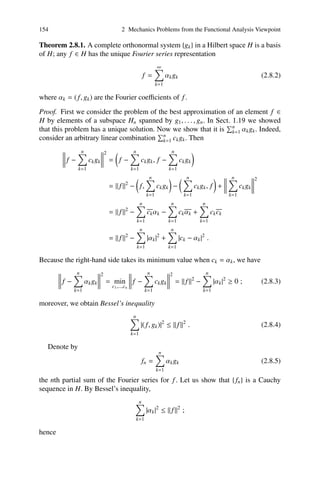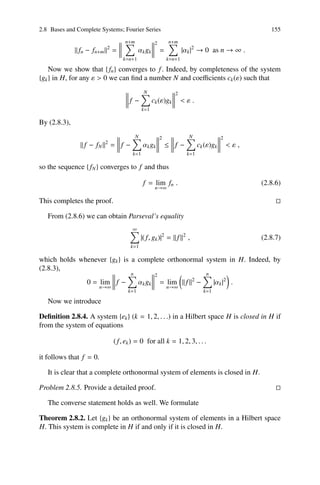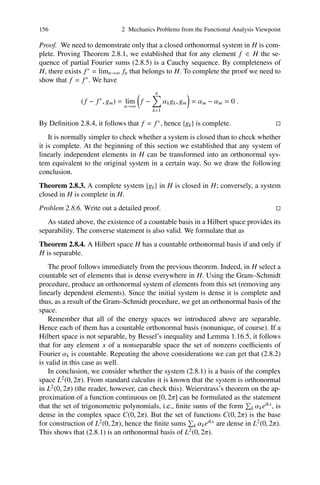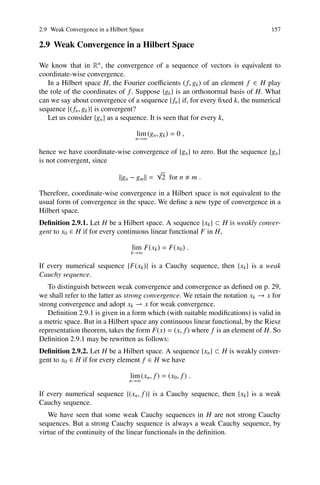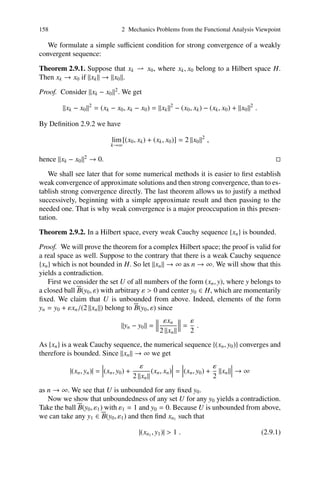Anzeige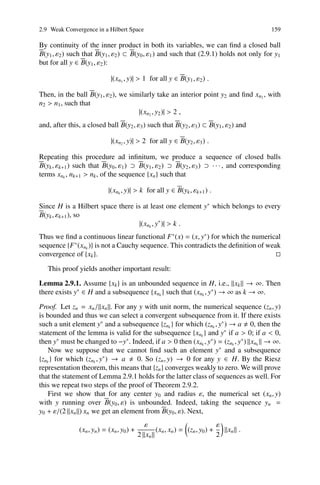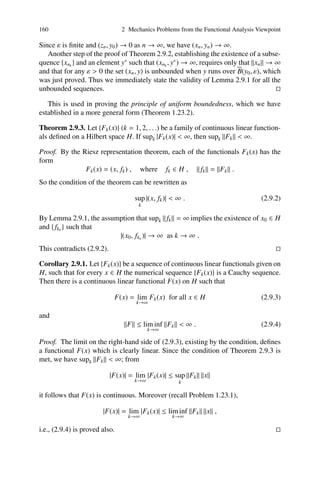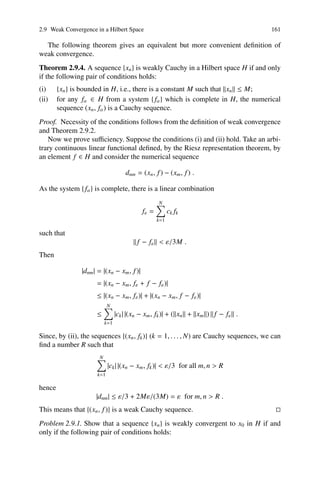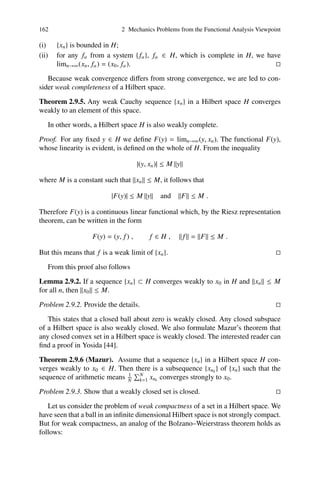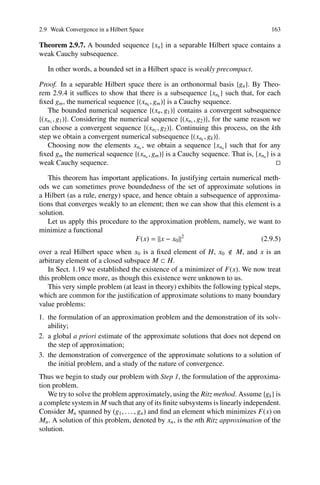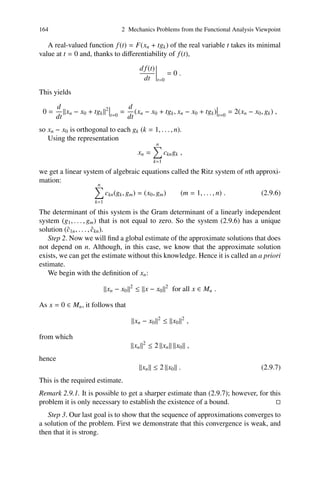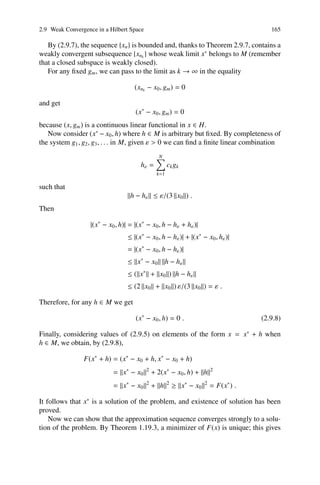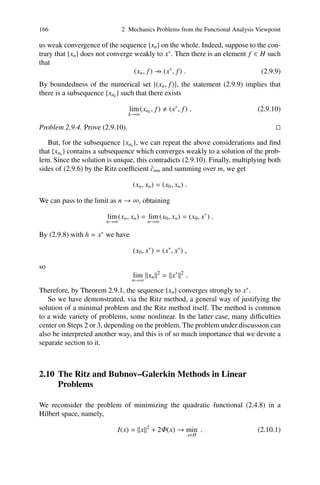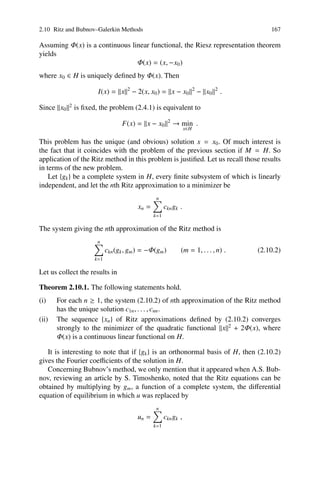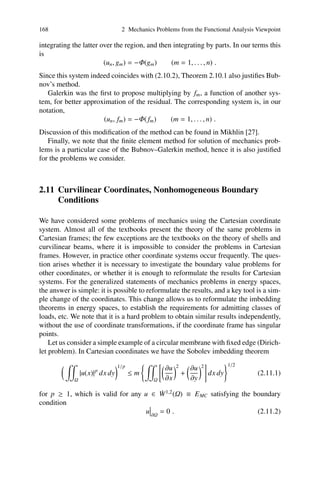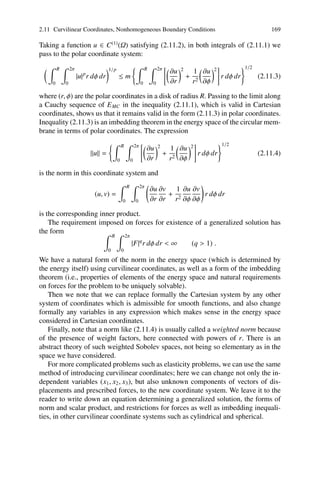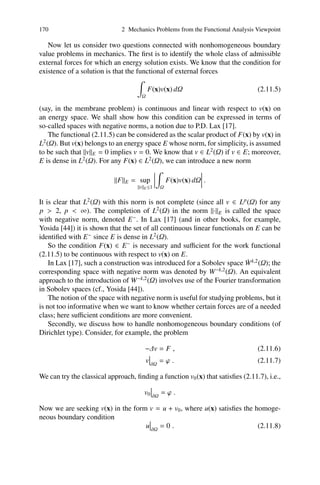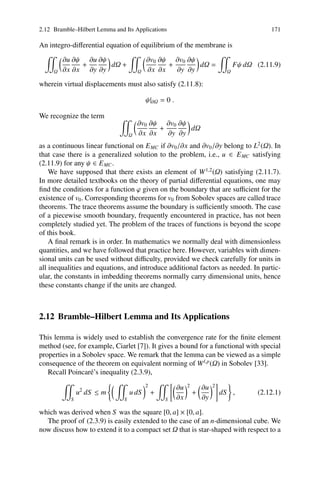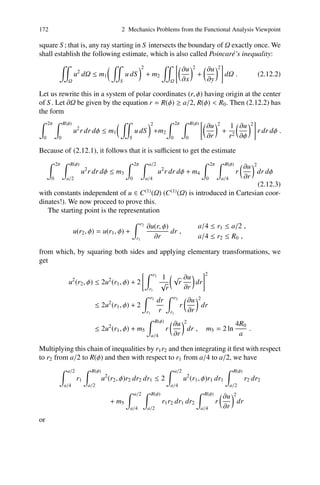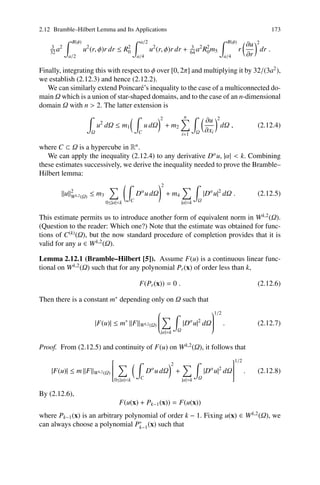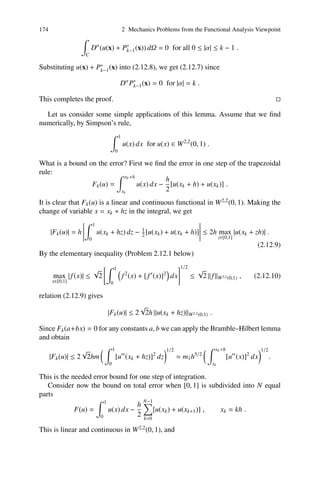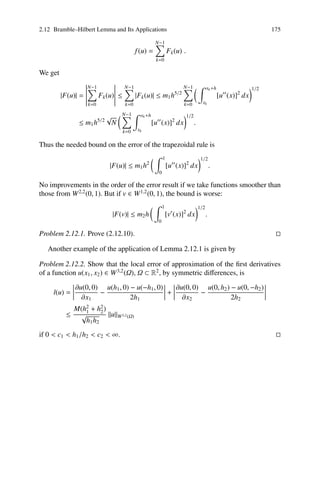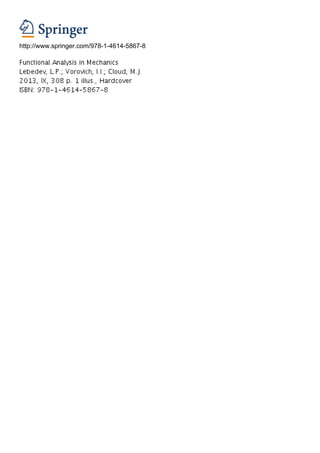Nächste SlideShare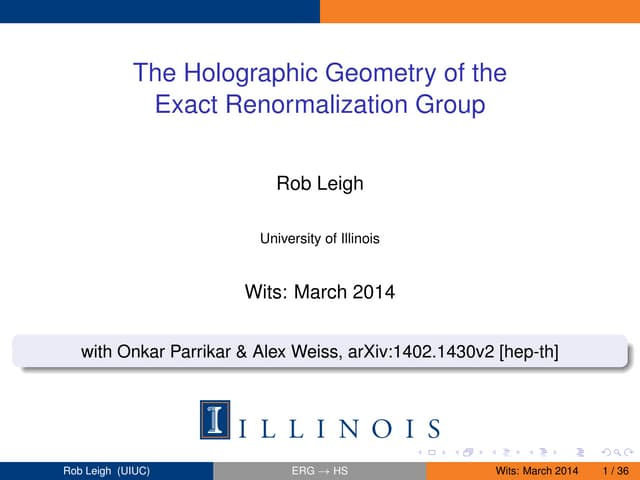Prof. Rob Leigh (University of Illinois)
1 von 62
Anzeige

### Functional analysis in mechanics 2e

1. Chapter 2 Mechanics Problems from the Functional Analysis Viewpoint In the past, an engineer could calculate mechanical stresses and strains using a pen- cil and a logarithmic slide rule. Modern mechanical models, on the other hand, are nonlinear, and even the linear models are complicated. Numerical methods in struc- tural dynamics cannot be applied without computers running specialized programs. However, a researcher should have a solid grasp of the equations that underlie a numerical model and the types of results that can be expected. New models ap- pear in mechanics on a regular basis. Some of these, when written out in detail, can span multiple pages and are clearly beyond pencil-and-paper approaches. Although functional analysis does not provide a detailed picture of the results to be expected from a complicated model, it can answer questions regarding whether the problem is mathematically well-posed (e.g., whether a solution exists and is unique). It may also indicate whether the results can be obtained by a general computer program or whether a special program, based on a knowledge of the general properties of the model, is required. In other words, functional analysis can provide valuable insight even to those who rely heavily on numerical approaches. 2.1 Introduction to Sobolev Spaces In his famous book , S.L. Sobolev (1908–1989) introduced some normed spaces that now bear his name; they are denoted by W m,p (Ω). The norm in W m,p (Ω) is ⎛ ⎞1/p ⎜ ⎜ ⎜ ⎟ ⎟ ⎟ u =⎜ ⎜ ⎜ ⎝ |D u| dΩ⎟ , α p ⎟ ⎟ ⎠ (2.1.1) Ω |α|≤m where m is an integer, p ≥ 1, and Ω is compact in Rn . (The Dα notation was in- troduced on p. 19.) Indeed this is a norm on the set of functions that possess con- tinuous derivatives up to order m on Ω, with satisfaction of axiom N3 ensured by Minkowski’s inequality (1.3.6). The completion of the resulting normed space is the Banach space W m,p (Ω). L.P. Lebedev et al., Functional Analysis in Mechanics, Springer Monographs in Mathematics, 115 DOI 10.1007/978-1-4614-5868-5_2, © Springer Science+Business Media, LLC 2013
2. 116 2 Mechanics Problems from the Functional Analysis Viewpoint For Ω a segment [a, b], the spaces W m,p (a, b) were introduced by Stefan Banach (1892–1945) in his dissertation. Our interest in Sobolev spaces is clear, as the ele- ments of each of our energy spaces will belong to W m,2 (Ω) for some m. Problem 2.1.1. (a) Demonstrate that if f (x) ∈ W 1,2 (a, b) and g(x) ∈ C (1) (a, b), then f (x)g(x) ∈ W 1,2 (a, b). (b) Show that A f (x) = f (x) deﬁnes a bounded linear operator acting from W 1,2 (0, 1) to L2 (0, 1). Generalized Notions of Derivative. For u ∈ L p (Ω), K.O. Friedrichs (1901–1982)  introduced the notion of strong derivative, calling v ∈ L p (Ω) a strong derivative Dα (u) if there is a sequence {ϕn } ⊂ C (∞) (Ω) such that |u(x) − ϕn (x)| p dΩ → 0 and |v(x) − Dα ϕn (x)| p dΩ → 0 as n → ∞ . Ω Ω Since C (∞) (Ω) is dense in any C (k) (Ω), an element of W m,p (Ω) has all strong deriva- tives up to the order m lying in L p (Ω). Another version of generalized derivative was proposed by Sobolev. He used, along with the classical integration by parts formula, an idea from the classical cal- culus of variations: if u(x) ϕ(x) dΩ = 0 Ω for all inﬁnitely diﬀerentiable functions ϕ(x) having compact support in an open domain Ω, then u(x) = 0 almost everywhere (everywhere if u(x) is to be continuous). We say that a function ϕ(x), inﬁnitely diﬀerentiable in Ω, has compact support in Ω ⊂ Rn if the closure of the set M = {x ∈ Ω : ϕ(x) 0} is a compact set in Ω. In the sense introduced by Sobolev, v ∈ L p (Ω) is called a weak derivative Dα u of u ∈ L p (Ω) if for every inﬁnitely diﬀerentiable function ϕ(x) with compact support in Ω we have u(x) Dα ϕ(x) dΩ = (−1)|α| v(x) ϕ(x) dΩ . (2.1.2) Ω Ω The two deﬁnitions of generalized derivative are equivalent . The proof would exceed the scope of our presentation, and the same is true for some other facts of this section. Imbedding Theorems. The results presented next are particular cases of Sobolev’s imbedding theorem. Later we will give proofs of some particular results when study- ing certain energy spaces for elastic models which turn out to be subspaces of Sobolev spaces. The theorems are formulated in terms of imbedding operators, a notion elucidated further in Sect. 2.2. We assume that the compact set Ω ⊂ Rn satisﬁes the cone condition. This means there is a ﬁnite circular cone in Rn such that any point of the boundary of Ω can be touched by the vertex of the cone while the cone lies fully inside Ω. This is the
3. 2.1 Introduction to Sobolev Spaces 117 condition under which Sobolev’s imbedding theorem is proved. We denote by Ωr an r-dimensional piecewise smooth hypersurface in Ω. (This means that, at any point of smoothness, in a local coordinate system, it is described by functions having all derivatives continuous up to order m locally, if we consider W m,p (Ω).) The theory of Sobolev spaces is a substantial branch of mathematics (see Adams , Lions and Magenes , etc.). We formulate only what is needed for our pur- poses. This is Sobolev’s imbedding theorem with some extensions: Theorem 2.1.1 (Sobolev–Kondrashov). The imbedding operator of the Sobolev space W m,p (Ω) to Lq (Ωr ) is continuous if one of the following conditions holds: (i) n > mp, r > n − mp, q ≤ pr/(n − mp); (ii) n = mp, q is ﬁnite with q ≥ 1. If n < mp, then the space W m,p (Ω) is imbedded into the H¨ lder space H α (Ω) when o α ≤ (mp − n)/p, and the imbedding operator is continuous. The imbedding operator of W m,p (Ω) to Lq (Ωr ) is compact (i.e., takes every bounded set of W m,p (Ω) into a precompact set of the corresponding space1 ) if (i) n > mp, r > n − mp, q < pr/(n − mp) or (ii) n = mp and q is ﬁnite with q ≥ 1. If n < mp then the imbedding operator is compact to H α (Ω) when α < (mp − n)/p. Note that this theorem provides imbedding properties not only for functions but also for their derivatives: u ∈ W m,p (Ω) =⇒ Dα u ∈ W m−k,p (Ω) for |α| = k . Also available are stricter results on the imbedding of Sobolev spaces on Ω into function spaces given on manifolds Ωr of dimension less than n; however, such trace theorems require an extended notion of Sobolev spaces. Let us formulate some useful consequences of Theorem 2.1.1. Theorem 2.1.2. Let γ be a piecewise diﬀerentiable curve in a compact set Ω ⊂ R2 . For any ﬁnite q ≥ 1, the imbedding operator of W 1,2 (Ω) to the spaces Lq (Ω) and Lq (γ) is continuous (and compact), i.e., max{ u Lq (Ω) , u Lq (γ) } ≤m u W 1,2 (Ω) (2.1.3) with a constant m which does not depend on u(x). Theorem 2.1.3. Let Ω ⊂ R2 be compact. If α ≤ 1, the imbedding operator of W 2,2 (Ω) to H α (Ω) is continuous; if α < 1, it is compact. For the ﬁrst derivatives, the imbedding operator to Lq (Ω) and Lq (γ) is continuous (and compact) for any ﬁnite q ≥ 1. 1 The notion of compact operator will be explored in Chap. 3.
4. 118 2 Mechanics Problems from the Functional Analysis Viewpoint Theorem 2.1.4. Let γ be a piecewise smooth surface in a compact set Ω ⊂ R3 . The imbedding operator of W 1,2 (Ω) to Lq (Ω) when 1 ≤ q ≤ 6, and to L p (γ) when 1 ≤ p ≤ 4, is continuous; if 1 ≤ q < 6 or 1 ≤ p < 4, respectively, then it is compact. We merely indicate how such theorems are proved. We will establish similar re- sults for the beam problem (see (2.3.5)) and for the clamped plate problem (see (2.3.21)) via integral representations of functions from certain classes. In like man- ner, Sobolev’s original proof is given for Ω a union of bounded star-shaped domains. A domain is called star-shaped with respect to a ball B if any ray with origin in B intersects the boundary of the domain only once. For a domain Ω which is bounded and star-shaped with respect to a ball B, a function u(x) ∈ C (m) (Ω) can be repre- sented in the form α α u(x) = x1 1 · · · xn n Kα (y) u(y) dΩ |α|≤m−1 B 1 + Kα (x, y) Dα u(y) dΩy (2.1.4) Ω |x − y|n−m |α|=m where Kα (y) and Kα (x, y) are continuous functions. Investigating properties of the integral terms on the right-hand side of the representation (2.1.4), Sobolev formu- lated his results; later they were extended to more general domains. Another method is connected with the Fourier transformation of functions. In the case of W m,2 (Ω), it is necessary to extend functions of C (m) (Ω) outside Ω in such a way that they belong to C m (Rn ) and W m,2 (Rn ). Then using the Fourier transforma- tion u(y) = (2π)−n/2 ˆ e−ix·y u(x) dx1 · · · dxn Rn along with the facts that u(x) L2 (Rn ) = u(y) ˆ L2 (Rn ) and Dα u(x) = (iy1 )α1 · · · (iyn )αn u(y) ˆ for u ∈ L2 (Rn ), we can present the norm in W m,2 (Ω) in the form u(x) 2 W m,2 (Rn ) = yα1 · · · yαn u(y) 1 n ˆ 2 L2 (Rn ) . |α|≤m We can then study the properties of the weighted space Lw (Rn ); this transformed 2 problem is simpler, as many of the problems involved are algebraic estimates of Fourier images. Moreover, we can consider W p,2 (Rn ) with fractional indices p. These lead to nec- essary and suﬃcient conditions for the trace problem: given W m,2 (Ω), ﬁnd the space W p,2 (∂Ω) in which W m,2 (Ω) is continuously imbedded. The inverse trace problem is, given W p,2 (Ω), ﬁnd the maximal index m such that every element u ∈ W p,2 (∂Ω)
5. 2.2 Operator of Imbedding 119 can be extended to Ω, u∗ ∈ W m,2 (Ω), in such a way that u∗ W m,2 (Ω) ≤c u W p,2 (∂Ω) . In this way, many results from the contemporary theory of elliptic (and other types of) equations and systems are obtained. We should mention that the trace theorems are formulated mostly for smooth manifolds, hence are not applicable to practical problems involving domains with corners. As stated in Lemma 1.16.3, all Sobolev spaces are separable. The same holds for all the energy spaces we introduce. 2.2 Operator of Imbedding Let Ω be a Jordan measurable compact set in Rn . Any element of C (k) (Ω) also be- longs to C(Ω). This correspondence — between an element of the space C (k) (Ω) and the same element in the space C(Ω) — is a linear operator. Although it resembles an identity operator, its domain and range diﬀer and it is called the imbedding operator from C (k) (Ω) to C(Ω). Clearly, C (k) (Ω) is a proper subset of C(Ω); this situation is typical for an imbedding operator that gives the correspondence between the same elements but considered in diﬀerent spaces. For a function u that belongs to both spaces, we have u C(Ω) ≤ u C (k) (Ω) , which shows that the imbedding operator is bounded or continuous (what can be said about its norm?). Moreover, by a consequence of Arzel` ’s theorem, it is compact. a We get a similar imbedding operator when considering the spaces p and q for 1 ≤ p < q. As (1.4.3) states, any element x of p belongs to q and x q ≤ c1 x p . Here and below, the constants ck are independent of the element of the spaces, so the imbedding operator from p to q is bounded. We encounter another imbedding operator when considering the correspondence between the same equivalence classes in the spaces L p (Ω) and Lq (Ω): if 1 ≤ p < q, then any element u of Lq (Ω) belongs to L p (Ω) and u L p (Ω) ≤ c2 u Lq (Ω) . So the imbedding operator from Lq (Ω) to L p (Ω) is also bounded. (Note that the relations between p and q are diﬀerent in the spaces r and Lr (Ω).) The situation is slightly diﬀerent when we consider the relation between the spaces C(Ω) and L p (Ω). It is clear that any function u from C(Ω) has a ﬁnite norm in L p (Ω). To use the imbedding idea, we identify the function u with the equivalence class from L p (Ω) that contains the stationary sequence (u, u, . . .), and in this way
6. 120 2 Mechanics Problems from the Functional Analysis Viewpoint consider u as an element of L p (Ω). Because u dΩ ≤ c3 u C(Ω) , Ω the imbedding operator from C(Ω) to L p (Ω) is bounded for any 1 ≤ p < ∞. This practice of identiﬁcation must be extended when we consider imbedding operators involving Sobolev spaces as treated in this book. In the imbedding from W 1,2 (Ω) to L p (Ω), the elements of W 1,2 (Ω) — the equivalence classes of functions from C (1) (Ω) — are diﬀerent from the equivalence classes from C(Ω) that constitute L p (Ω); however, each class from C (1) (Ω) is contained by some class from C(Ω), and we identify these classes. In this sense, we say that W 1,2 (Ω) is imbedded into L p (Ω) and the imbedding operator is continuous (or even compact). The imbedding of a Sobolev space into the space of continuous functions is even more complicated, although the general ideas are the same. We will consider this in more detail when dealing with the energy space for a plate. In this case we cannot directly identify an element of a Sobolev space, which is an equivalence class, with a continuous function. However, we observe that for a class of equivalent sequences in the norm of a Sobolev space, for any sequence that enters into the class, there is a limit element, a continuous function that does not diﬀer for other representative sequences, and we identify the whole class with this function. The inequality relat- ing the Sobolev norm of the class and the continuous norm of this function states that this identiﬁcation or correspondence is a bounded operator whose linearity is obvious. Some other inequalities state that the operator is compact. 2.3 Some Energy Spaces A Beam. Earlier we noted that the set S of all real-valued continuous functions y(x) having continuous ﬁrst and second derivatives on [0, l] and satisfying the boundary conditions y(0) = y (0) = y(l) = y (l) = 0 (2.3.1) is a metric space under the metric l 1/2 1 d(y, z) = B(x)[y (x) − z (x)]2 dx . (2.3.2) 2 0 We called this an energy space for the clamped beam. We can introduce an inner product 1 l (y, z) = B(x)y (x)z (x) dx (2.3.3) 2 0 and norm l 1/2 1 y = B(x)[y (x)]2 dx 2 0
7. 2.3 Some Energy Spaces 121 such that d(y, z) = y − z . But this space is not complete (it is clear that there are Cauchy sequences whose limits do not belong to C (2) (0, l); the reader should construct an example). To have a complete space, we must apply the completion theorem. The actual energy space denoted by E B is the completion of S in the metric (2.3.2) (or, what amounts to the same thing, in the inner product (2.3.3)). Let us consider some properties of the elements of E B . An element y(x) ∈ E B is a set of Cauchy sequences equivalent in the metric (2.3.2). Let {yn (x)} be a represen- tative sequence of y(x). Then, according to (2.3.2), l 1/2 1 B(x)[yn (x) − ym (x)]2 dx → 0 as n, m → ∞ . 2 0 If we assume that 0 < m1 ≤ B(x) ≤ m2 , then the sequence of second derivatives {yn (x)} is a Cauchy sequence in the norm of L2 (0, l) because l l m1 [yn (x) − ym (x)]2 dx ≤ B(x)[yn (x) − ym (x)]2 dx . 0 0 Hence {yn (x)} is a representative sequence of some element of L2 (0, l), and we can regard y ∈ Eb as having a second derivative that belongs to L2 (0, l). Now consider {yn (x)}. For any y(x) ∈ S we get x y (x) = y (t) dt . 0 So for a representative {yn (x)} of a class y(x) ∈ E B we have x l |yn (x) − ym (x)| ≤ |yn (t) − ym (t)| dt ≤ 1 · |yn (t) − ym (t)| dt 0 0 l 1/2 ≤ l1/2 [yn (x) − ym (x)]2 dx 0 l 1/2 ≤ (l/m1 )1/2 B(x)[yn (x) − ym (x)]2 dx 0 → 0 as n, m → ∞ . (2.3.4) It follows that {yn (x)} converges uniformly on [0, l]; hence there exists a limit func- tion z(x) which is continuous on [0, l]. This function does not depend on the choice of representative sequence (Problem 2.3.1 below). The same holds for a sequence of functions {yn (x)}: its limit is a function y(x) continuous on [0, l]. Moreover, y (x) = z(x) .
8. 122 2 Mechanics Problems from the Functional Analysis Viewpoint To prove this, it is necessary to repeat the arguments of Sect. 1.11 on the diﬀeren- tiability of the elements of C (k) (Ω), with due regard for (2.3.4). From (2.3.4) and the similar inequality for {yn (x)} we get l 1/2 1 max |y(x)| + |y (x)| ≤ m B(x)[y (x)]2 dx (2.3.5) x∈[0,l] 2 0 for some constant m independent of y(x) ∈ E B . So each element y(x) ∈ E B can be identiﬁed with an element y(x) ∈ C (1) (0, l) in such a way that (2.3.5) holds. This correspondence is an imbedding operator, and we interpret (2.3.5) as a statement that the imbedding operator from E B to C (1) (0, l) is continuous (cf., Sect. 1.21). Henceforth we refer to the elements of E B as if they were continuously diﬀerentiable functions, attaching the properties of the uniquely determined limit functions to the corresponding elements of E B themselves. Problem 2.3.1. Prove that the function z(x), discussed above, is independent of the choice of representative sequence. We are interested in analyzing all terms that appear in the statement of the equi- librium problem for a body as a minimum potential energy problem. So we will consider the functional giving the work of external forces. For the beam, it is l A= F(x)y(x) dx . 0 This is well-deﬁned on E B if F(x) ∈ L(Ω); moreover, (2.3.5) shows that the work of external forces can contain terms of the form [Fk y(xk ) + Mk y (xk )] , k which can be interpreted as the work of point forces and point moments, respec- tively. This is a consequence of the continuity of the imbedding from E B to C (1) (0, l). Remark 2.3.1. Alternatively we can deﬁne E B on a base set S 1 of smoother func- tions, in C (4) (0, l) say, satisfying (2.3.1). The result is the same, since S 1 is dense in S with respect to the norm of C (2) (0, l). However, sometimes such a deﬁnition is convenient. Remark 2.3.2. Readers familiar with the contemporary literature in this area may have noticed that Western authors usually deal with Sobolev spaces, studying the properties of operators corresponding to problems under consideration; we prefer to deal with energy spaces, studying ﬁrst their properties and then those of the corre- sponding operators. Although these approaches lead to the same results, in our view the physics of a particular problem should play a principal role in the analysis — in this way the methodology seems simpler, clearer, and more natural. In papers de- voted to the study of elastic bodies, we mainly ﬁnd interest in the case of a clamped boundary. Sometimes this is done on the principle that it is better to deal solely with
9. 2.3 Some Energy Spaces 123 homogeneous Dirichlet boundary conditions, but often it is an unfortunate conse- quence of the use of the Sobolev spaces H k (Ω). The theory of these spaces is well developed but is not amenable to the study of other boundary conditions. Success in the investigation of mechanics problems can be much more diﬃcult without the beneﬁt of the physical ideas brought out by the energy spaces. Remark 2.3.3. In deﬁning the energy space of the beam, we left aside the question of smoothness of the stiﬀness function B(x). From a mathematical standpoint this is risky since, in principle, B(x) can be nonintegrable. But in the case of an actual physical beam, B(x) can have no more than a ﬁnite number of discontinuities and must be diﬀerentiable everywhere else. For simplicity, we shall continue to make realistic assumptions concerning physical parameters such as stiﬀness and elastic constants; in particular, we shall suppose whatever degree of smoothness is required for our purposes. A Membrane (Clamped Edge). The subset of C (1) (Ω) consisting of all functions satisfying u(x, y) ∂Ω = 0 (2.3.6) with the metric 1/2 ∂u ∂v 2 ∂u ∂v 2 d(u, v) = − + − dx dy (2.3.7) Ω ∂x ∂x ∂y ∂y is an incomplete metric space. If we introduce an inner product ∂u ∂v ∂u ∂v (u, v) = + dx dy (2.3.8) Ω ∂x ∂x ∂y ∂y consistent with (2.3.7), we get an inner product space. Its completion in the metric (2.3.7) is the energy space E MC for the clamped membrane, a real Hilbert space. What can we say about the elements U(x, y) ∈ E MC ? If {un (x, y)} is a representa- tive of U(x, y), then 1/2 ∂un ∂um 2 ∂un ∂um 2 − + − dx dy → 0 as n, m → ∞ . Ω ∂x ∂x ∂y ∂y and we see that the sequences of ﬁrst derivatives {∂un /∂x}, {∂un /∂y} are Cauchy sequences in the norm of L2 (Ω). What about U(x, y) itself? If we extend each un (x, y) by zero outside Ω, we can write x ∂un (s, y) un (x, y) = ds 0 ∂s (assuming, without loss of generality, that Ω is conﬁned to the band 0 ≤ x ≤ a). Squaring both sides and integrating over Ω, we get
10. 124 2 Mechanics Problems from the Functional Analysis Viewpoint 2 x ∂un (s, y) u2 (x, y) dx dy = ds dx dy Ω n Ω 0 ∂s 2 a ∂un (s, y) ≤ 1· ds dx dy Ω 0 ∂s a ∂un (s, y) 2 ≤ a ds dx dy Ω 0 ∂s ∂un (x, y) 2 ≤ a2 dx dy . Ω ∂x This means that if {∂un /∂x} is a Cauchy sequence in the norm of L2 (Ω), then so is {un }. Hence we can consider elements U(x, y) ∈ E MC to be such that U(x, y), ∂U/∂x, and ∂U/∂y belong to L2 (Ω). As a consequence of the last chain of inequalities, we get Friedrichs’ inequality ∂U 2 ∂U 2 U 2 (x, y) dx dy ≤ m + dx dy Ω Ω ∂x ∂y which holds for any U(x, y) ∈ E MC and a constant m independent of U(x, y). Membrane (Free Edge). Although it is natural to introduce the energy space using the energy metric (2.3.7), we cannot distinguish between two states u1 (x, y) and u2 (x, y) of the membrane with free edge if u2 (x, y) − u1 (x, y) = c = constant . This is the only form of “rigid” displacement possible for a membrane. We ﬁrst show that no other rigid displacements (i.e., displacements associated with zero strain energy) are possible. The proof is a consequence of Poincar´ ’s inequality e 2 ∂u 2 ∂u 2 u2 dx dy ≤ m u dx dy + + dx dy (2.3.9) Ω Ω Ω ∂x ∂y for a function u(x, y) ∈ C (1) (Ω). The constant m does not depend on u(x, y). Proof of Poincar´ ’s inequality . We ﬁrst assume that Ω is the square [0, a] × [0, a] e and write down the identity x2 ∂u(s, y1 ) y2 ∂u(x2 , t) u(x2 , y2 ) − u(x1 , y1 ) = ds + dt . x1 ∂s y1 ∂t Squaring both sides and then integrating over the square, ﬁrst with respect to the variables x1 and y1 and then with respect to x2 and y2 , we get
11. 2.3 Some Energy Spaces 125 u2 (x2 , y2 ) − 2u(x2 , y2 )u(x1 , y1 ) + u2 (x1 , y1 ) dx1 dy1 dx2 dy2 Ω Ω 2 x2 ∂u(s, y1 ) y2 ∂u(x2 , t) = ds + dt dx1 dy1 dx2 dy2 Ω Ω x1 ∂s y1 ∂t 2 a ∂u(s, y1 ) a ∂u(x2 , t) ≤ 1· ds + 1· dt dx1 dy1 dx2 dy2 Ω Ω 0 ∂s 0 ∂t a ∂u(s, y1 ) 2 a ∂u(x2 , t) 2 ≤ 2a ds + dt dx1 dy1 dx2 dy2 Ω Ω 0 ∂s 0 ∂t a a ∂u 2 ∂u 2 ≤ 2a4 + dx dy . 0 0 ∂x ∂y Note that, along with the Schwarz inequality for integrals, we have used the elemen- tary inequality (a + b)2 ≤ 2(a2 + b2 ) which follows from the fact that (a − b)2 ≥ 0. The beginning of this chain of inequalities is 2 a2 u2 (x, y) dx dy − 2 u(x, y) dx dy + a2 u2 (x, y) dx dy Ω Ω Ω so 2 ∂u 2 ∂u 2 2a2 u2 dx dy ≤ 2 u dx dy + 2a4 + dx dy Ω Ω Ω ∂x ∂y and we get (2.3.9) with m = max(a2 , 1/a2 ). It can be shown that Poincar´ ’s inequality holds on more general domains. A e modiﬁcation of (2.3.9) will appear in Sect. 2.12. Let us return to the free membrane problem. Provided we consider only the mem- brane’s state of stress, any two states are identical if they are described by functions u1 (x, y) and u2 (x, y) whose diﬀerence is constant. We gather all functions (such that the diﬀerence between any two is a constant) into a class denoted by u∗ (x, y). There is a unique representative of u∗ (x, y) denoted by ub (x, y) such that ub (x, y) dx dy = 0 . (2.3.10) Ω For this balanced representative (or balanced function), Poincar´ ’s inequality be- e comes ∂ub 2 ∂ub 2 u2 (x, y) dx dy ≤ m + dx dy . (2.3.11) Ω b Ω ∂x ∂y As the right-hand side is zero for a “rigid” displacement, so is the left-hand side and it follows that the balanced representative associated with a rigid displacement must be zero. Hence u(x, y) = c is the only permissible form for a rigid displacement of a membrane.
12. 126 2 Mechanics Problems from the Functional Analysis Viewpoint Because (2.3.11) has the same form as Friedrichs’ inequality, we can repeat our former arguments to construct the energy space E MF for a free membrane using the balanced representatives of the classes u∗ (x, y). In what follows we shall use this space E MF , remembering that its elements satisfy (2.3.10). The condition (2.3.10) is a geometrical constraint resulting from our mathemat- ical technique. Solving the static free membrane problem, we must remember that the formulation of the equilibrium problem does not impose this constraint — the membrane can move as a “rigid body” in the direction normal to its own surface. But if we consider only deformations and the strain energy deﬁned by the ﬁrst par- tial derivatives of u(x, y), the results must be independent of such motions. Consider then the functional of the work of external forces A= F(x, y)U(x, y) dx dy . Ω If we use the space E MF , then A makes sense if F(x, y) ∈ L2 (Ω) (guaranteed by (2.3.11) together with the Schwarz inequality in L2 (Ω)). This is the only restriction on external forces for a clamped membrane. However, in the case of equilibrium for a free membrane, the functional A must be invariant under transformations of the form u(x, y) → u(x, y) + c with any constant c. This requires F(x, y) dx dy = 0 . (2.3.12) Ω Again, we consider the equilibrium problem where rigid motion, however, is possi- ble. Since we did not introduce inertia forces, we have formally equated the mass of the membrane to zero. In this situation of zero mass, any forces with nonzero resul- tant would make the membrane as a whole move with inﬁnite acceleration. Thus, (2.3.12) also precludes such physical nonsense. Meanwhile, Sobolev’s imbedding theorem permits us to incorporate forces ψ acting on the boundary S of Ω into the functional describing the work of external forces: F(x, y)u(x, y) dx dy + ψu ds . (2.3.13) Ω S In this case, the condition of invariance of the functional under constant motions yields the condition F(x, y) dx dy + ψ ds = 0 . (2.3.14) Ω S Note that in the formulation of the Neumann problem, this quantity ψ appears in the boundary condition: ∂u =ψ. ∂n S The reader may ask why, if the membrane can move as a rigid body, it cannot rotate freely in the manner of an ordinary free rigid body. The answer is that in this model, unlike the other models for elastic bodies in this book, rotation of the mem-
13. 2.3 Some Energy Spaces 127 brane as a rigid body alters the membrane strain energy. In the membrane model, the deﬂection u(x, y) is the deﬂection that is imposed on the prestressed state of the membrane. The membrane equilibrium problem is an example of a problem for a prestressed body. There is another way to formulate the equilibrium problem for a free mem- brane, based on a diﬀerent method of introducing the energy space. Let us return to Poincar´ ’s inequality (2.3.9). Denote e 2 1/2 u 1 = u dx dy + D(u) Ω where ∂u 2 ∂u 2 D(u) = + dx dy , Ω ∂x ∂y and recall that the norm in W 1,2 (Ω) is 2 1/2 u W 1,2 (Ω) = u2 dx dy + D(u) . Ω By (2.3.9), the norms · 1 and · W 1,2 (Ω) are equivalent on the set of continuously diﬀerentiable functions, and hence on W 1,2 (Ω). Let us use the norm · 1 on W 1,2 (Ω). Now the space W 1,2 (Ω) is the completion, with respect to the norm · 1 , of the functions continuously diﬀerentiable on Ω. Let us take an element U(x, y) ∈ W 1,2 (Ω) and select from U(x, y) an arbitrary representative Cauchy sequence {uk (x, y)}. A smooth function uk (x, y) can be uniquely written in the form uk (x, y) = uk (x, y) + ak ˜ where uk dx dy = 0 . ˜ Ω Since 2 uk − um 2 1 = (ak − am ) dx dy + D(˜ k − um ) → 0 as k, m → ∞ , u ˜ Ω we see that {ak } is a numerical Cauchy sequence that has a limit corresponding to U. This means that {ak } belongs to c, the space of numerical sequences each of which has a limit. It is easy to show that this limit does not depend on the choice of representative sequence {uk (x, y)}, and we can regard it as a rigid displacement of the membrane. Now, because the membrane is not geometrically ﬁxed and can be moved through any uniform displacement with no change in energy, we place all the elements of W 1,2 (Ω) that characterize a strained state of the membrane into the same class such that for any two elements U (x, y) and U (x, y) of the class and any representative sequences {uk (x, y)} and {uk (x, y)} taken from them, {˜ k (x, y)} and u {˜ k (x, y)} are equivalent in the norm (i.e., D(˜ k − uk ) → 0) and the diﬀerence se- u u ˜ quence {ak − ak } is in c. This construction of the classes is equivalent to the con- struction of the factor space W 1,2 (Ω)/c, which can also be called the energy space
14. 128 2 Mechanics Problems from the Functional Analysis Viewpoint for the free membrane. The zero of this energy factor space is the set of the elements of W 1,2 (Ω) each containing as a representative an element from c. Clearly if we wish to have the energy functional deﬁned in this space, the necessary condition for its continuity is that its linear part — the work of external forces — must be zero over any constant displacement. The latter involves the self-balance condition (2.3.12). It is seen that the principal part of any class-element of the new energy space, deﬁned by the sequences {˜ k (x, y)}, coincides with the corresponding sequences for the el- u ements of the space E MF ; moreover, this correspondence between the two energy spaces maintains equal norms in both spaces. So we can repeat the procedure to establish the existence-uniqueness theorem in the new energy space, using the proof in E MF . The restriction (2.3.12) (or (2.3.14)) is necessary for the functional of external forces to be uniquely deﬁned for an element U∗ (x, y). We shall use the same nota- tion E MF for this type of energy space since there is a one-to-one correspondence, preserving distances and inner products, between the two types of energy space for the free membrane. Moreover, we shall always make clear which version we mean. Those familiar with the theory of the Neumann problem for Laplace’s equation should note that the necessary condition for solvability that arises in mathematical physics as a mathematical consequence, i.e., ψ ds = 0 , S is a particular case of (2.3.14) when F(x, y) = 0. This means that for solvability of the problem, the external forces acting on the membrane edge should be self- balanced. Finally, we note that Poisson’s equation governs not only membranes, but also situations in electricity, magnetism, hydrodynamics, mathematical biology, and other ﬁelds. So we can consider spaces such as E M in various other sciences. It is clear that the results will be the same. We will proceed to introduce other energy spaces in a similar manner: they will be completions of corresponding metric (inner product) spaces consisting of smooth functions satisfying certain boundary conditions. The problem is to determine prop- erties of the elements of those completions. As a rule, metrics must contain all the strain energy terms (we now discuss only linear systems). For example, we can con- sider a membrane whose edge is elastically supported; then we must include the energy of elastic support in the expression for the energy metric. Bending a Plate. Here we begin with the work of internal forces on variations of displacements −(w1 , w2 ) = − Dαβγδ ργδ (w1 ) ραβ (w2 ) dx dy (2.3.15) Ω where w1 (x, y) is the normal displacement of the plate midsurface Ω, w2 (x, y) can be considered as its variation, ραβ (u) are components of the change-of-curvature tensor,
15. 2.3 Some Energy Spaces 129 ∂2 u ∂2 u ∂2 u ρ11 (u) = , ρ12 = , ρ22 = , ∂x2 ∂x∂y ∂y2 Dαβγδ are components of the tensor of elastic constants of the plate such that Dαβγδ = Dγδαβ = D βαγδ (2.3.16) and, for any symmetric tensor ραβ there exists a constant m0 > 0 such that 2 Dαβγδ ργδ ραβ ≥ m0 ρ2 . αβ (2.3.17) α,β=1 We suppose the Dαβγδ are constants, but piecewise continuity of these parameters would be suﬃcient. For the theory of shells and plates, Greek indices will assume values from the set {1, 2} while Latin indices will assume values from the set {1, 2, 3}. The repeated index convention for summation is also in force. For example, we have 2 aαβ bαβ ≡ aαβ bαβ . α,β=1 We ﬁrst consider a plate with clamped edge ∂Ω: ∂w w ∂Ω = =0. (2.3.18) ∂n ∂Ω (Of course, the variation of w must satisfy (2.3.18) as well.) Let us show that on S 4 , the subset of C (4) (Ω) consisting of those functions which satisfy (2.3.18), the form (w1 , w2 ) given in (2.3.15) is an inner product. We begin with the axiom P1: 2 (w, w) = Dαβγδ ραβ (w) ργδ (w) dx dy ≥ m0 ρ2 (w) dx dy αβ Ω Ω α,β=1 ∂2 w 2 ∂2 w 2 ∂2 w 2 = m0 +2 + dx dy ≥ 0 . Ω ∂x2 ∂x∂y ∂y2 If w = 0 then (w, w) = 0. If (w, w) = 0 then, on Ω, ∂2 w ∂2 w ∂2 w =0, =0, =0. ∂x2 ∂x∂y ∂y2 It follows that w(x, y) = a1 + a2 x + a3 y , where the ai are constants. By (2.3.18) then, w(x, y) = 0. Hence P1 is satisﬁed. Satisfaction of P2 follows from (2.3.16), and it is evident that P3 is also satisﬁed.
16. 130 2 Mechanics Problems from the Functional Analysis Viewpoint Thus S 4 with inner product (2.3.15) is an inner product space; its completion in the corresponding metric is the energy space E PC for a clamped plate. Let us consider some properties of the elements of E PC . It was shown that ∂2 w 2 ∂2 w 2 ∂2 w 2 2 m0 +2 + dx dy Ω ∂x2 ∂x∂y ∂y2 ≤ Dαβγδ ργδ (w) ραβ (w) dx dy ≡ (w, w) . (2.3.19) Ω From this and the Friedrichs inequality, written ﬁrst for w and then for the ﬁrst derivatives of w ∈ S 4 as well, we get ∂w 2 ∂w 2 w2 dx dy ≤ m1 + dx dy Ω Ω ∂x ∂y ∂2 w 2 ∂2 w 2 ∂2 w 2 ≤ m2 +2 + dx dy Ω ∂x2 ∂x∂y ∂y2 ≤ m3 Dαβγδ ργδ (w) ραβ (w) dx dy ≡ m3 (w, w) . (2.3.20) Ω Hence if {wn } ⊂ S 4 is a Cauchy sequence in E PC , then the sequences ∂wn ∂wn ∂2 wn ∂2 wn ∂2 wn {wn }, , , , , , ∂x ∂y ∂x2 ∂x∂y ∂y2 are Cauchy sequences in L2 (Ω). So we can say that an element W of the completion E PC is such that W(x, y) and all its derivatives up to order two are in L2 (Ω). We now investigate W(x, y) further. Let w ∈ S 4 and w(x, y) ≡ 0 outside Ω. Sup- pose Ω lies in the domain {(x, y) : x > 0, y > 0}. Then the representation x y ∂2 w(s, t) w(x, y) = ds dt 0 0 ∂s∂t holds. Using H¨ lder’s inequality and (2.3.20), we get o x y ∂2 w(s, t) ∂2 w(s, t) |w(x, y)| ≤ ds dt ≤ 1· ds dt 0 0 ∂s∂t Ω ∂s∂t ∂2 w(s, t) 2 1/2 ≤ (mes Ω)1/2 ds dt ≤ m4 (w, w)1/2 . (2.3.21) Ω ∂s∂t This means that if {wn } ⊂ S 4 is a Cauchy sequence in the metric of E PC , then it converges uniformly on Ω. Hence there exists a limit function w0 (x, y) = lim wn (x, y) n→∞
17. 2.3 Some Energy Spaces 131 which is continuous on Ω; this function is identiﬁed, as above, with the correspond- ing element of E PC and we shall say that E PC is continuously imbedded into C(Ω). The functional describing the work of external forces A= F(x, y) W(x, y) dx dy Ω now makes sense if F(x, y) ∈ L(Ω); moreover, it can contain the work of point forces F(xk , yk ) w0 (xk , yk ) k and line forces F(x, y) w0 (x, y) ds γ where γ is a line in Ω and w0 (x, y) is the corresponding limit function for W(x, y). Remark 2.3.4. Modern books on partial diﬀerential equations often require that F(x, y) ∈ H −2 (Ω). This is a complete characterization of external forces — how- ever, it is diﬃcult for an engineer to verify this property. Now let us consider a plate with free edge. In this case, we also wish to use the inner product (2.3.15) to create an energy space. As in the case of a membrane with free edge, the axiom P1 is not fulﬁlled: we saw that from (w, w) = 0 it follows that w(x, y) = a1 + a2 x + a3 y . (2.3.22) This admissible motion of the plate as a rigid whole is called a rigid motion, but still diﬀers from real “rigid” motions of the plate as a three-dimensional body. Poincar´ ’s inequality (2.3.9) implies that the zero element of the correspond- e ing completion consists of functions of the form (2.3.22). Indeed, taking w(x, y) ∈ C (4) (Ω) we write down Poincar´ ’s inequality for ∂w/∂x: e ∂w 2 ∂w 2 ∂2 w 2 ∂2 w 2 dx dy ≤ m dx dy + + dx dy , Ω ∂x Ω ∂x Ω ∂x2 ∂x∂y and then the same inequality for ∂w/∂y with the roles of x and y interchanged. From these and (2.3.9) we get ∂w 2 ∂w 2 2 w2 + + dx dy ≤ m1 w dx dy Ω ∂x ∂y Ω ∂w 2 ∂w 2 + dx dy + dx dy Ω ∂x Ω ∂y ∂w 2 2 ∂w2 2 ∂2 w 2 + +2 + dx dy . Ω ∂x2 ∂x∂y ∂y2 From (2.3.19) it follows that
18. 132 2 Mechanics Problems from the Functional Analysis Viewpoint ∂w 2 ∂w 2 2 w2 + + dx dy ≤ m2 w dx dy Ω ∂x ∂y Ω ∂w 2 ∂w 2 + dx dy + dx dy Ω ∂x Ω ∂y + Dαβγδ ργδ (w) ραβ (w) dx dy . (2.3.23) Ω For any function w(x, y) ∈ C (4) (Ω), we can take suitable constants ai and ﬁnd a function wb (x, y) of the form wb (x, y) = w(x, y) + a1 + a2 x + a3 y (2.3.24) such that ∂wb ∂wb wb dx dy = 0 , dx dy = 0 , dx dy = 0 . (2.3.25) Ω Ω ∂x Ω ∂y As for the membrane with free edge, we can now consider a subset S 4b of C (4) (Ω) consisting of balanced functions satisfying (2.3.25). We construct an energy space E PF for a plate with free edge as the completion of S 4b in the metric induced by the inner product (2.3.15). From (2.3.25), (2.3.23), and (2.3.19), we see that an element W(x, y) ∈ E PF is such that W(x, y) and all its “derivatives” up to order two are in L2 (Ω). We could show the existence of a limit function w0 (x, y) = lim wn ∈ C(Ω) n→∞ for any Cauchy sequence {wn }, but in this case the technique is more complicated and, in what follows, we have this result as a particular case of the Sobolev imbed- ding theorem. Note that (2.3.25) can be replaced by w(x, y) dx dy = 0 , x w(x, y) dx dy = 0 , y w(x, y) dx dy = 0 , Ω Ω Ω since these also uniquely determine the ai for a class of functions of the form (2.3.24). (This possibility follows from Sobolev’s general result  on equivalent norms in Sobolev spaces.) The system (2.3.25) represents constraints that are absent in nature. For a static problem, there must be a certain invariance of some objects under transformations of the form (2.3.24) with arbitrary constants ak . In particular, the work of external forces should not depend on the ak if the problem is stated correctly. This leads to the necessary conditions
19. 2.3 Some Energy Spaces 133 F(x, y) dx dy = 0 , x F(x, y) dx dy = 0 , y F(x, y) dx dy = 0 . Ω Ω Ω (2.3.26) The mechanical sense of (2.3.26) is clear: the resultant force and moments must vanish. This is the condition for a self-balanced force system. Problem 2.3.2. What is the form of (2.3.26) if the external forces contain point and line forces? An energy space for a free plate, as for the membrane with free edge, can be introduced in another way: namely, we begin with an element of W 2,2 (Ω), selecting a representative sequence {wk (x, y)+ak +bk x+ck y}, where w satisﬁes (2.3.25) and ak , ˜ ˜ bk , ck are constants. It is easy to show that the numerical sequences {ak }, {bk }, {ck } are Cauchy and therefore belong to the space c. Next we combine into a class-element all the elements of W 2,2 (Ω) whose diﬀerences are a linear polynomial a + bx + cy, and state that the energy space is the factor space of W 2,2 (Ω) by the space which is the completion of the space of linear polynomials with respect to the norm a + bx + cy = (a2 + b2 + c2 )1/2 . In the factor space, the zero is the class of all Cauchy sequences whose diﬀer- ences are equivalent (in the norm of W 2,2 (Ω)) to a sequence {ak + bk x + ck y} with {ak }, {bk }, {ck } ∈ c. The norm of W, an element in the factor space, is 1/2 Dαβγδ ργδ (W) ραβ (W) dx dy . Ω The elements of the factor space are uniquely identiﬁed with the elements of the energy space E PF whose elements satisfy (2.3.25). Moreover, the identiﬁcation is isometric so, as for the membrane, we can use this factor space as the energy space and repeat the proof of the existence-uniqueness theorem in terms of the elements of the energy factor space. The self-balance condition for the external forces is a nec- essary condition to have the functional of the work of external forces be meaningful. The reader may also consider mixed boundary conditions: how must the treatment be modiﬁed if the plate is clamped only along a segment AB ⊂ Ω so that w(x, y) AB = 0 , with the rest of the boundary free of geometrical constraints? Linear Elasticity. We return to the problem of linear elasticity, considered in Sect. 1.5. Let us introduce a functional describing the work of internal forces on variations v(x) of the displacement ﬁeld u(x): −(u, v) = − ci jkl kl (u) i j (v) dΩ . (2.3.27) Ω For the notation, see (1.5.7)–(1.5.9). We recall that the strain energy E4 (u) of an elastic body occupying volume Ω is related to the introduced inner product as fol-
20. 134 2 Mechanics Problems from the Functional Analysis Viewpoint lows: (u, u) = u 2 = 2E4 (u) . i jkl The elastic moduli c may be piecewise continuous functions satisfying (1.5.8) and (1.5.9), which guarantee that all inner product axioms are satisﬁed by (u, v) for vector functions u, v continuously diﬀerentiable on Ω, except P1: from (u, u) = 0 it follows that u = a + b × x. Note that (u, v) is consistent with the metric (1.5.10). Let us consider boundary conditions prescribed by u(x) ∂Ω = 0 . (2.3.28) If we use the form (2.3.27) on the set S 3 of vector-functions u(x) satisfying (2.3.28) and such that each of their components is of class C (2) (Ω), then (u, v) is an inner product and S 3 with this inner product becomes an inner product space. Its com- pletion E EC in the corresponding metric (or norm) is the energy space of an elastic body with clamped boundary. To describe the properties of the elements of E EC , we establish Korn’s inequality. Lemma 2.3.1 (Korn). For a vector function u(x) ∈ S 3 , we have 3 ∂ui 2 |u|2 + dΩ ≤ m ci jkl kl (u) i j (u) dΩ Ω i, j=1 ∂x j Ω for some constant m which does not depend on u(x). Proof. By (1.5.9) and Friedrichs’ inequality, it is suﬃcient to show that 3 3 ∂ui 2 dΩ ≤ m1 2 i j (u) dΩ . Ω i, j=1 ∂x j Ω i, j=1 i≤ j Consider the term on the right: 3 3 1 ∂ui ∂u j 2 A≡ 2 i j (u) dΩ = + dΩ Ω i, j=1 4 Ω i, j=1 ∂x j ∂xi i≤ j i≤ j ⎧ ⎫ ⎪ ⎪ 3 ⎪ ⎪ ⎪ ⎪ ⎪ ⎪ ⎨ ∂ui 2 1 3 ∂ui 2 ∂u j 2 ⎪ ∂ui ∂u j ⎪ ⎬ = ⎪ ⎪ + + +2 ⎪ dΩ . ⎪ ⎪ i=1 ∂xi Ω⎪ 4 i, j=1 ∂x j ∂xi ∂x j ∂xi ⎪ ⎪ ⎪ ⎪ ⎩ ⎭ i< j Integrating by parts (twice) the term 3 3 1 ∂ui ∂u j 1 ∂ui ∂u j B≡ dΩ = dΩ 2 Ω i, j=1 ∂x j ∂xi 2 Ω i, j=1 ∂xi ∂x j i< j i< j
21. 2.4 Generalized Solutions in Mechanics 135 and using the elementary inequality |ab| ≤ (a2 + b2 )/2, we get 3 3 1 ∂ui 2 ∂u j 2 1 ∂ui 2 |B| ≤ + dΩ = dΩ . 4 Ω i, j=1 ∂xi ∂x j 2 Ω i=1 ∂xi i< j Therefore 3 1 ∂ui 2 A≥ dΩ , 4 Ω i, j=1 ∂x j which completes the proof. By Korn’s inequality, each component of an element U ∈ E EC belongs to E MC , i.e., the ui and their ﬁrst derivatives belong to L2 (Ω). Note that the construction of an energy space is the same if the boundary condi- tion (2.3.28) is given only on some part ∂Ω1 of the boundary of Ω: u(x) ∂Ω = 0 . 1 Korn’s inequality also holds, but its proof is more complicated (see, for example, [26, 11]). If we consider an elastic body with free boundary, we encounter issues similar to those for a membrane or plate with free edge: we must circumvent the diﬃculty with the zero element of the energy space. The restrictions u dΩ = 0 , x × u(x) dΩ = 0 , (2.3.29) Ω Ω provide that the rigid motion u = a + b × x becomes zero, and that Korn’s inequality remains valid for smooth vector functions satisfying (2.3.29). So by completion, we get an energy space E EF with known properties: all Cartesian components of vectors pertain to the space W 1,2 (Ω). As for a free membrane, we can also organize an energy space of classes — a factor space — in which the zero element is the set of all elements whose diﬀerences between any representative sequences in the norm of (W 1,2 (Ω))3 are equivalent to a sequence of the form {ak + bk × x} such that the Cartesian components of the vectors {ak } and {bk } constitute some elements of the space c. 2.4 Generalized Solutions in Mechanics We now discuss how to introduce generalized solutions in mechanics. We begin with Poisson’s equation −Δu(x, y) = F(x, y) , (x, y) ∈ Ω , (2.4.1)
22. 136 2 Mechanics Problems from the Functional Analysis Viewpoint where Ω is a bounded open domain in R2 . The Dirichlet problem consists of this equation supplemented by the boundary condition u ∂Ω = 0 . (2.4.2) Let u(x, y) be its classical solution; i.e., let u ∈ C (2) (Ω) satisfy (2.4.1) and (2.4.2). Let ϕ(x, y) be a function with compact support in Ω. Again, this means that ϕ ∈ C (∞) (Ω) and the closure of the set M = {(x, y) ∈ Ω : ϕ(x, y) 0} lies in Ω. Multiplying both sides of (2.4.1) by ϕ(x, y) and integrating over Ω, we get − ϕ(x, y) Δu(x, y) dx dy = F(x, y) ϕ(x, y) dx dy . (2.4.3) Ω Ω If this equality holds for every inﬁnitely diﬀerentiable function ϕ(x, y) with compact support in Ω, and if u ∈ C (2) (Ω) and satisﬁes (2.4.2), then, as is well known from the classical calculus of variations, u(x, y) is the unique classical solution to the Dirichlet problem. But using (2.4.3), we can pose this Dirichlet problem directly without using the diﬀerential equation (2.4.1); namely, u(x, y) is a solution to the Dirichlet problem if, obeying (2.4.2), it satisﬁes (2.4.3) for every ϕ(x, y) that is inﬁnitely diﬀerentiable with compact support in Ω. If F(x, y) belongs to L p (Ω) then we can take, as it seems, u(x, y) having second derivatives in the space L p (Ω); such a u(x, y) is not a classical solution, and it is natural to call it a generalized solution. We can go further by applying integration by parts to the left-hand side of (2.4.3) as follows: ∂u ∂ϕ ∂u ∂ϕ + dx dy = F(x, y) ϕ(x, y) dx dy . (2.4.4) Ω ∂x ∂x ∂y ∂y Ω In such a case we may impose weaker restrictions on a solution u(x, y) and call it the generalized solution if it belongs to E MC , the energy space for a clamped membrane. Equation (2.4.4) deﬁnes this solution if it holds for every ϕ(x, y) that has a compact support in Ω. Note the disparity in requirements on u(x, y) and ϕ(x, y). Further integration by parts on the left-hand side of (2.4.4) yields − u(x, y) Δϕ(x, y) dx dy = F(x, y) ϕ(x, y) dx dy . (2.4.5) Ω Ω Now we can formally consider solutions from the space L(Ω) and this is a new class of generalized solutions. This way leads to the theory of distributions originated by Schwartz . He extended the notion of generalized solution to a class of linear continuous function- als, or distributions, deﬁned on the set D(Ω) of all functions inﬁnitely diﬀerentiable in Ω and with compact support in Ω. For this it is necessary to introduce the con- vergence and other structures of continuity in D(Ω). Unfortunately D(Ω) is not a normed space (see, for example, Yosida  — it is a locally convex topological space) and its presentation would exceed our scope. This theory justiﬁes, in particu-
23. 2.4 Generalized Solutions in Mechanics 137 lar, the use of the so-called δ-function, which was introduced in quantum mechanics via the equality ∞ δ(x − a) f (x) dx = f (a) , (2.4.6) −∞ valid for every continuous function f (x). Physicists considered δ(x) to be a function vanishing everywhere except at x = 0, where its value is inﬁnity. Any known theory of integration gave zero for the value of the integral on the left-hand side of (2.4.6), and the theory of distributions explained how to understand such strange functions. It is interesting to note that the δ-function was well known in classical mechanics, too; if we consider δ(x − a) as a unit point force applied at x = a, then the integral on the left-hand side of (2.4.6) is the work of this force on the displacement f (a), which is indeed f (a). So we have several generalized statements of the Dirichlet problem, but which one is most natural from the viewpoint of mechanics? From mechanics it is known that a solution to the problem is a minimizer of the total potential energy functional ∂u 2 ∂u 2 I(u) = + dx dy − 2 Fu dx dy . (2.4.7) Ω ∂x ∂y Ω According to the calculus of variations, a minimizer of I(u) on the subset of C (2) (Ω) consisting of all functions satisfying (2.4.2), if it exists, is a classical solution to the Dirichlet problem. But we also can consider I(u) on the energy space E MC if F(x, y) ∈ L p (Ω) for p > 1. Indeed, the ﬁrst term in I(u) is well-deﬁned in E MC and can be written in the form u 2 ; the second, Φ(u) = − F(x, y) u(x, y) dx dy , Ω is a linear functional with respect to u(x, y). It is also continuous in E MC ; by virtue of H¨ lder’s inequality with exponents p and q = p/(p − 1), we have o 1/p 1/q Fu dx dy ≤ |F| p dx dy |u|q dx dy Ω Ω Ω ≤ m1 F L p (Ω) u W 1,2 (Ω) ≤ m2 u E MC . To show this, we have used the imbedding Theorem 2.1.2 and the Friedrichs in- equality. By Theorem 1.21.1, Φ(u) is continuous in E MC , and therefore so is I(u). Thus I(u) is of the form I(u) = u 2 + 2Φ(u) . (2.4.8) Let u0 ∈ E MC be a minimizer of I(u), i.e., I(u0 ) ≤ I(u) for all u ∈ E MC . (2.4.9)
24. 138 2 Mechanics Problems from the Functional Analysis Viewpoint We try a method from the classical calculus of variations. Take u = u0 + v where v is an arbitrary element of E MC . Then I(u) = I(u0 + v) = u0 + v 2 + 2Φ(u0 + v) = (u0 + v, u0 + v) + 2Φ(u0 + v) = u0 2 + 2 (u0 , v) + 2 v 2 + 2Φ(u0 ) + 2 Φ(v) = u0 2 + 2Φ(u0 ) + 2 [(u0 , v) + Φ(v)] + 2 v 2 . From (2.4.9), we get 2 [(u0 , v) + Φ(v)] + 2 v 2 ≥0. Since is an arbitrary real number (in particular it can take either sign, and the ﬁrst term, if nonzero, dominates for small in magnitude), it follows that (u0 , v) + Φ(v) = 0 . (2.4.10) In other words, ∂u0 ∂v ∂u0 ∂v + dx dy − F(x, y) v(x, y) dx dy = 0 . (2.4.11) Ω ∂x ∂x ∂y ∂y Ω This equality holds for every v ∈ E MC and deﬁnes the minimizer u0 ∈ E MC . Note that (2.4.11) has the same form as (2.4.4). So we have introduced a notion of generalized solution that has an explicit me- chanical background. Deﬁnition 2.4.1. An element u ∈ E MC is called the generalized solution to the Dirichlet problem if u satisﬁes (2.4.11) for any v ∈ E MC . We can also obtain (2.4.11) from the principle of virtual displacements (work). This asserts that in the state of equilibrium, the sum of the work of internal forces (which is now the variation of the strain energy with a negative sign) and the work of external forces is zero on all virtual (admissible) displacements. In the case under consideration, both approaches to introducing generalized en- ergy solutions are equivalent. In general, however, this is not so, and the virtual work principle has wider applicability. If F(x, y) is a nonconservative load depending on u(x, y), we cannot use the principle of minimum total energy; however, (2.4.11) re- mains valid since it has the mathematical form of the virtual work principle. In what follows, we often use this principle to pose problems in equation form. Since the part of the presentation from (2.4.8) up to (2.4.10) is general and does not depend on the speciﬁc form (2.4.11) of the functional I(u), we can formulate Theorem 2.4.1. Let u0 be a minimizer of a functional I(u) = u 2 +2Φ(u) given in an inner product (Hilbert) space H, where the functional Φ(u) is linear and continuous. Then u0 satisﬁes (2.4.10) for every v ∈ H.
25. 2.4 Generalized Solutions in Mechanics 139 Equation (2.4.10) is a necessary condition for minimization of the functional I(u), analogous to the condition that the ﬁrst derivative of an ordinary function must vanish at a point of minimum. We can obtain (2.4.10) formally by evaluating d I(u0 + v) =0. (2.4.12) d =0 This is valid for the following reason. Given u0 and v, the functional I(u0 + v) is an ordinary function of the numerical variable , and assumes a minimum value at = 0. The left-hand side of (2.4.12) can be interpreted as a partial derivative at u = u0 in the direction v, and is called the Gˆ teaux derivative of I(u) at u = u0 in a the direction of v. We shall return to this issue later. The Dirichlet problem for a clamped membrane is a touchstone for all static prob- lems in continuum mechanics. In a similar way we can introduce a natural notion of generalized solution for other problems under consideration. As we said, each of them can be represented as a minimization problem for a total potential energy functional of the form (2.4.10) in an energy space. For example, equation (2.4.11), a particular form of (2.4.10) for a clamped membrane, is the same for a free mem- brane — we need only replace E MC by E MF . The quantity Φ(u), to be a continuous linear functional in E MF , must be supplemented with self-balance condition (2.3.12) for the load. Let us concretize equation (2.4.10) for each of the other problems we have under consideration. Plate. The deﬁnition of generalized solution w0 ∈ E P is given by the equation Dαβγδ ργδ (w0 ) ραβ (w) dx dy − F(x, y) w(x, y) dx dy Ω Ω m − Fk w(xk , yk ) − f (s) w(x, y) ds = 0 (2.4.13) k=1 γ (see the notation of Sect. 2.3) which must hold for every w ∈ E P . The equation is the same for any kind of homogeneous boundary conditions (i.e., for usual ones) but the energy space will change from one set of boundary conditions to another. If a plate can move as a rigid whole, the requirement that F(x, y) ∈ L(Ω) , f (s) ∈ L(γ) , for Φ to be a continuous linear functional, must be supplemented with self-balance conditions for the load: m F(x, y) wi (x, y) dx dy + Fk wi (xk , yk ) + f (s) wi (x, y) ds = 0 (2.4.14) Ω k=1 γ
26. 140 2 Mechanics Problems from the Functional Analysis Viewpoint for i = 1, 2, 3, where w1 (x, y) = 1, w2 (x, y) = x, and w3 (x, y) = y. This condition is necessary if we use the space where the set of rigid plate motions is the zero of the space. If from each element of the energy space we select a representative us- ing (2.3.25), then on the energy space of all representatives with norm · P we can prove existence and uniqueness of the energy solution without self-balance condi- tion (2.4.14). But for solvability of the initial problem for a free plate, we still should require (2.4.14) to hold as constraints (2.3.25) are absent in the problem statement. Note that for each concrete problem we must specify the energy space. The same is true for the following problem. Linear Elasticity. The generalized solution u is deﬁned by the integro-diﬀerential equation ci jkl kl (u) i j (v) dΩ − F(x, y, z) · v(x, y, z) dΩ Ω Ω − f(x, y, z) · v(x, y, z) dS = 0 , (2.4.15) Γ where F and f are forces distributed over Ω and over some surface Γ ⊂ Ω, respec- tively. If we consider the Dirichlet (or ﬁrst) problem of elasticity, which is u(x) ∂Ω = 0 where ∂Ω is the boundary of Ω, the solution u should belong to the space E EC and equation (2.4.15) must hold for every virtual displacement v ∈ E EC . Note that in this case, f(x, y, z) · v(x, y, z) dS = 0 . ∂Ω The load, thanks to Theorem 2.1.4 and Korn’s inequality, is of the class Fi (x, y, z) ∈ L6/5 (Ω) , fi (x, y, z) ∈ L4/3 (Γ) (i = 1, 2, 3) , where Fi (x, y, z) and fi are Cartesian components of F and f, respectively, and Γ is a piecewise smooth surface in Ω. This provides continuity of Φ(w). In the second problem of elasticity there are given forces distributed over the boundary: ci jkl kl (x) n j (x) ∂Ω = fi (x) , where the n j are Cartesian components of the unit exterior normal to ∂Ω. This can be written in tensor notation as σ(x) · n ∂Ω = f(x) where the components of stress tensor σ are related to the components of the strain tensor by σi j = ci jkl kl . As for equilibrium problems for free membranes and plates, in the case of a free elastic body we must require that the load be self-balanced:
27. 2.5 Existence of Energy Solutions to Some Mechanics Problems 141 F(x) dΩ + f(x) dS = 0 , Ω Γ x × F(x) dΩ + x × f(x) dS = 0 . (2.4.16) Ω Γ The solution is required to be in E EF . We have argued that it is legitimate to introduce the generalized solution in such a way. Of course, full legitimacy will be assured when we prove that this solution exists and is unique in the corresponding space. We emphasize once more that the deﬁnition of generalized solution arose in a natural way from the variational principle of mechanics. 2.5 Existence of Energy Solutions to Some Mechanics Problems In Sect. 2.4 we introduced generalized solutions for several mechanics problems and reduced those problems to a solution of the abstract equation (u, v) + Φ(v) = 0 (2.5.1) in an energy (Hilbert) space. We obtained some restrictions on the forces to provide continuity of the linear functional Φ(v) in the energy space. The following theorem guarantees solvability of those mechanics problems in a generalized sense. Theorem 2.5.1. Assume Φ(v) is a continuous linear functional given on a Hilbert space H. Then there is a unique element u ∈ H that satisﬁes (2.5.1) for every v ∈ H. Proof. By the Riesz representation theorem there is a unique u0 ∈ H such that the continuous linear functional Φ(v) is represented in the form Φ(v) = (v, u0 ) ≡ (u0 , v). Hence (2.5.1) takes the form (u, v) + (u0 , v) = 0 . (2.5.2) We need to ﬁnd u ∈ H that satisﬁes (2.5.2) for every v ∈ H. Rewriting it in the form (u + u0 , v) = 0 , we see that its unique solution is u = −u0 . This theorem answers the question of solvability, in the generalized sense, of the problems treated in Sect. 2.4. To demonstrate this, we rewrite Theorem 2.5.1 in concrete terms for a pair of problems. Theorem 2.5.2. Assume F(x, y) ∈ L(Ω) and f (x, y) ∈ L(γ) where Ω ⊂ R2 is com- pact and γ is a piecewise smooth curve in Ω. The equilibrium problem for a plate with clamped edge has a unique generalized solution: there is a unique w0 ∈ E PC which satisﬁes (2.4.13) for all w ∈ E PC .
28. 142 2 Mechanics Problems from the Functional Analysis Viewpoint Changes for a plate which is free of clamping are evident: we must add the self- balance condition (2.4.14) for forces and replace the space E PC by E PF . Theorem 2.5.3. Assume all Cartesian components of the volume forces F(x, y, z) are in L6/5 (Ω) and those of the surface forces f(x, y, z) are in L4/3 (S ), where Ω is compact in R3 and S is a piecewise smooth surface in Ω. Then the problem of equilibrium of an elastic body occupying Ω, with clamped boundary, has a unique generalized solution u ∈ E EC ; namely, u(x, y, z) satisﬁes (2.4.15) for every v ∈ E EC . In both theorems, the load restrictions provide continuity of the corresponding functionals Φ, the work of external forces. Problem 2.5.1. Formulate existence theorems for the other mechanics problems dis- cussed in Sect. 2.4. 2.6 Operator Formulation of an Eigenvalue Problem We have seen how to use the Riesz representation theorem to prove the existence and uniqueness of a generalized solution. Now let us consider another application of the Riesz representation theorem: how to cast a problem as an operator equation. The eigenvalue equation for a membrane has the form ∂ 2 u ∂2 u + = −λu . (2.6.1) ∂x2 ∂y2 Similar to the equilibrium problem for a membrane, we can introduce a general- ized solution to the eigenvalue problem for a clamped membrane by the integro- diﬀerential equation ∂u ∂v ∂u ∂v + dx dy = λ uv dx dy . (2.6.2) Ω ∂x ∂x ∂y ∂y Ω The eigenvalue problem is to ﬁnd a nontrivial element u ∈ E MC and a corresponding number λ such that u satisﬁes (2.6.2) for every v ∈ E MC . First we reformulate this problem as an operator equation u = λKu (2.6.3) in the space E MC . For this, consider the term F(v) = uv dx dy Ω as a functional in E MC , with respect to v, when u is a ﬁxed element of E MC . It is seen that F(v) is a linear functional. By the Schwarz inequality
29. 2.6 Operator Formulation of an Eigenvalue Problem 143 1/2 1/2 |F(v)| = uv dx dy ≤ u2 dx dy v2 dx dy Ω Ω Ω hence by the Friedrichs inequality |F(v)| ≤ m u v = m1 v (2.6.4) (hereafter the norm · and the inner product (·, ·) are taken in E MC ). So F(v) is a continuous linear functional acting in the Hilbert space E MC . By the Riesz represen- tation theorem, F(v) has the unique representation F(v) ≡ uv dx dy = (v, f ) = ( f, v) . (2.6.5) Ω What have we shown? For every u ∈ E MC , by this representation, there is a unique element f ∈ E MC . Hence the correspondence u→ f is an operator f = K(u) from E MC to E MC . Let us display some properties of this operator. First we show that it is linear. Let f1 = K(u1 ) and f2 = K(u2 ) . Then (λ1 u1 + λ2 u2 )v dx dy = (K(λ1 u1 + λ2 u2 ), v) Ω while on the other hand, (λ1 u1 + λ2 u2 )v dx dy = λ1 u1 v dx dy + λ2 u2 v dx dy Ω Ω Ω = λ1 (K(u1 ), v) + λ2 (K(u2 ), v) = (λ1 K(u1 ) + λ2 K(u2 ), v) . Combining these we have (K(λ1 u1 + λ2 u2 ), v) = (λ1 K(u1 ) + λ2 K(u2 ), v) , hence K(λ1 u1 + λ2 u2 ) = λ1 K(u1 ) + λ2 K(u2 ) because v ∈ E MC is arbitrary. Therefore linearity is proven. Now let us rewrite (2.6.4) in terms of this representation: |(K(u), v)| ≤ m u v . Take v = K(u); then
30. 144 2 Mechanics Problems from the Functional Analysis Viewpoint K(u) 2 ≤ m u K(u) and it follows that K(u) ≤ m u . (2.6.6) Hence K is a continuous operator in E MC . Equation (2.6.2) can now be written in the form (u, v) = λ(K(u), v) . Since v is an arbitrary element of E MC , this equation is equivalent to the operator equation u = λK(u) with a continuous linear operator K. By (2.6.6), we get λK(u) − λK(v) = |λ| K(u − v) ≤ m |λ| u − v . If m |λ| < 1 , then λK is a contraction operator in E MC and, by the contraction mapping principle, there is a unique ﬁxed point of λK which clearly is u = 0. So the set |λ| < 1/m does not contain real eigenvalues of the problem. Further, we shall see (and this is well known in mechanics) that eigenvalues in this problem must be real. The fact that the set |λ| < 1/m does not contain real eigenvalues, and so any eigenvalues of the problem, has a clear mechanical sense: the lowest eigenfrequency of oscillation of a bounded clamped membrane is strictly positive. Note that from (2.6.2), when v = u it follows that an eigenvalue must be positive. In a similar way, we can introduce eigenvalue problems for plates and elastic bodies. Here we can obtain corresponding equations of the form (2.6.3) with con- tinuous linear operators and can also show that the corresponding lowest eigenvalues are strictly positive. All this we leave to the reader; later we shall consider eigen- value problems in more detail. In what follows, we shall see that, using the Riesz representation theorem, one can also introduce operators and operator equations for nonlinear problems of me- chanics. One of them is presented in the next section. 2.7 Problem of Elastico-Plasticity; Small Deformations Following the lines of a paper by I.I. Vorovich and Yu.P. Krasovskij  that was published in a sketchy form, we consider a variant of the theory of elastico-plasticity (Il’yushin ), and justify the method of elastic solutions for corresponding bound- ary value problems.
Anzeige